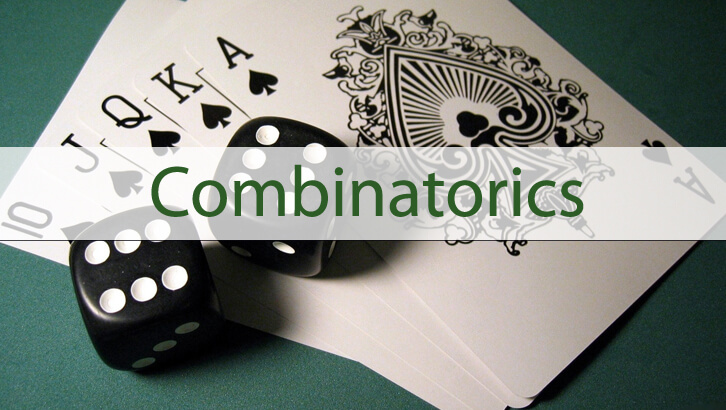# Permutations and Combinations

Question
Sum of three whole numbers a, b and c is 10. How many ordered triplets (a, b, c) exist? (This is a variant on the previous question that can be found here). Will post the solution in a day or two.Correct Answer
12C2

Explanatory Answer

a + b + c = 10. a,b,c are whole numbers. Now this is similar to the previous question that we solved by placing 10 sticks and simplifying. The discussion can be seen here .

We cannot follow a similar approach as a,b,c can be zero. Let us modify the approach a little bit. Let us see if we can remove the constraint that a,b,c can be zero.

If we give a minimum of 1 to a,b,c then the original approach can be used. And then we can finally remove 1 from each of a,b, c. So, let us distribute 13 sticks across a, b and c and finally remove one from each.

a + b + c = 13. Now, let us place ten sticks in a row

|     |     |     |    |     |     |     |     |     |     |     |     |

This question now becomes the equivalent of placing two ‘+’ symbols somewhere between these sticks. For instance

|     |     |     | + |     |     |     |     |  + |     |     |     |, This would be the equivalent of 4 + 5 + 4. or, a = 4, b = 5, c = 4.

There are 12 slots between the sticks, out of which one has to select 2 for placing the ‘+’s.

The number of ways of doing this is 12C2.